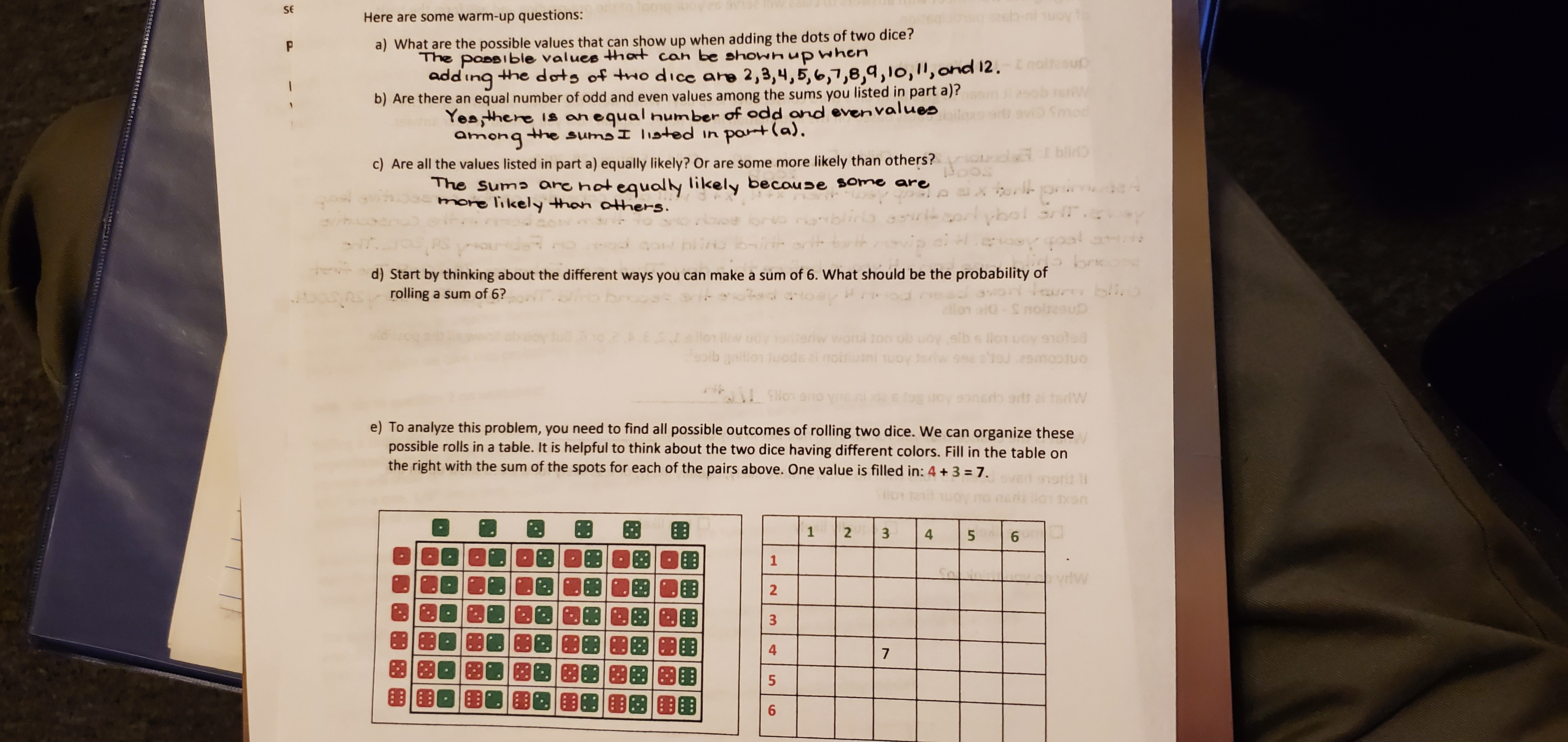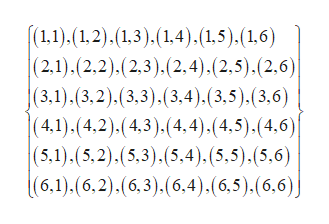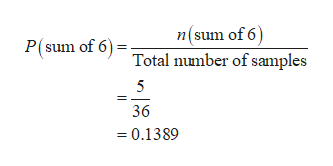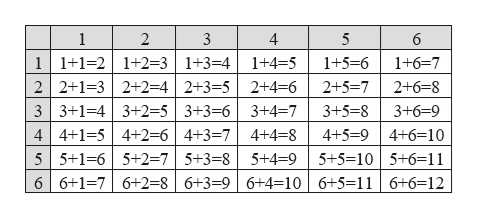.SEHere are some warm-up questions:Q: Aonk ua) What are the possible values that can show up when adding the dots of two dice?The possible values thort can be showh up whenadd ing the dots of two dice are 2,3,4,5,6,7,8,9,10,,ond 12. uPb) Are there an equal number of odd and even values among the sums you listed in part a)?Yeathere is an equal humber of odd and even valuesamongthe sumsI listed in port(a),Smodc) Are all the values listed in part a) equally likely? Or are some more likely than others?CHI EProsThe Suma are hotequally likely because some aremore likely thon others.xtorl prg9uiobol srip ci e oy goolnanh-.9oM biino bi oth+Start by thinking about the different ways you can make a sum of 6. What should be the probability ofrolling a sum of 6?W01 chsou blio2ilonaid- \$noleeu3io2 # 8.5.Jwwotl ton ob OV.SIba lO1ucy 9101009btionuods anoini uoytsw 9ou 2smooJUOSo no yoe ni suov enaris 9rdtaitdWe) To analyze th is problem, you need to find all possible outcomes of rolling two dice. We can organize thesepossible rolls in a table. It is helpful to think about the two dice having different colors. Fill in the table onthe right with the sum of the spots for each of the pairs above. One value is filled in: 4+3 7.SXS1261dw4756

Question

I need help answering parts (d) and (e)help_outlineImage Transcriptionclose. SE Here are some warm-up questions: Q: Aonk u a) What are the possible values that can show up when adding the dots of two dice? The possible values thort can be showh up when add ing the dots of two dice are 2,3,4,5,6,7,8,9,10,,ond 12. u P b) Are there an equal number of odd and even values among the sums you listed in part a)? Yeathere is an equal humber of odd and even values amongthe sumsI listed in port(a), Smod c) Are all the values listed in part a) equally likely? Or are some more likely than others? CHI EPr os The Suma are hotequally likely because some are more likely thon others. xtorl pr g9uiobol sr ip ci e oy gool nanh -. 9oM biino bi oth + Start by thinking about the different ways you can make a sum of 6. What should be the probability of rolling a sum of 6? W01 chs ou blio 2ilonaid- \$noleeu 3io2 # 8.5.J wwotl ton ob OV.SIba lO1ucy 910100 9btionuods anoini uoytsw 9ou 2smooJUO So no yoe ni suov enaris 9rdtaitdW e) To analyze th is problem, you need to find all possible outcomes of rolling two dice. We can organize these possible rolls in a table. It is helpful to think about the two dice having different colors. Fill in the table on the right with the sum of the spots for each of the pairs above. One value is filled in: 4+3 7. SXS 1 2 6 1 dw 4 7 5 6 fullscreen
Step 1

(d)

The probability of rolling a sum of 6 is 0.1389 and it is calculated below:

From the given information, two dice are rolled.

The total sample space is,help_outlineImage Transcriptionclose(1.1).(1.2).(1,3).(1,4).(1.5).(1,6) (2,1).(2,2).(2,3).(2,4).(2.5).(2,6) |(3,1).(3,2).(3,3).(3,4). (3,5). (3,6) (4,1).(4,2).(4,3).(4.4).(4,5).(4,6)| (5,1),(5,2).(5,3).(5,4), (5,5).(5,6) (6,1).(6,2).(6,3).(6,4), (6,5).(6,6)j fullscreen
Step 2

The total numbers samples are 36.

The sample space for sum of 6 is {(1,5),(2,4),(3,3),(4,2),(5,1)}. The number of samples is 5.help_outlineImage Transcriptionclosen(sum of 6) Total number of samples P(sum of 6) 5 36 =0.1389 fullscreen
Step 3

e)

The possible outcomes for adding th...help_outlineImage Transcriptionclose1 2 3 4 5 6 11+1-2 1+2=3 1+3=4 2 2+1-3 2+2=4 |2+3=5 | 2+4 6 3 3+1-43+2=5 3+3=6 3+4 7 44+1-54+2=64+3-74+4-8 55+1-6 5+2=75+3=8 5+4-95+5=10 5+6-11 6 6+1-76+2=8| 6+3=9 | 6+4 10 6+5=116+6=12 1+4 5 1+5-6 1+6-7 2+5-7 2+6 8 3+6=9 3+5=8 4+5=9 4+6=10 fullscreen

Want to see the full answer?

See Solution

Want to see this answer and more?

Our solutions are written by experts, many with advanced degrees, and available 24/7

See Solution
Tagged in

Other